# 逻辑回归

## 概述

Jerry 86 1
Tom 98 1
Lily 58 0
…… …… ……

### 逻辑函数

$y = h(w_1x_1 + w_2x_2 + w_3x_3 + ... + w_nx_n + b)$

$h= \frac{1}{1+e^{-t}}$

$\quad t=w^Tx+b$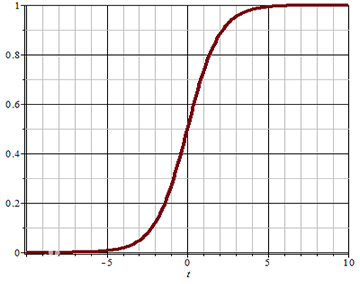### 分类问题的损失函数

$E(y, \hat{y}) = -[y \ log(\hat{y}) + (1-y)log(1-\hat{y})]$

• $y=1$时，预测值$\hat{y}$越接近于1，$log(\hat{y})$越接近于0，损失函数值越小，表示误差越小，预测的越准确；当预测时$\hat{y}$接近于0时，$log(\hat{y})$接近于负无穷大，加上符号后误差越大，表示越不准确；
• $y=0$时，预测值$\hat{y}$越接近于0，$log(1-\hat{y})$越接近于0，损失函数值越小，表示误差越小，预测越准确；当预测值$\hat{y}$接近于1时，$log(1-\hat{y})$接近于负无穷大，加上符号后误差越大，表示越不准确.

## 逻辑回归实现

sklearn中，逻辑回归相关API如下：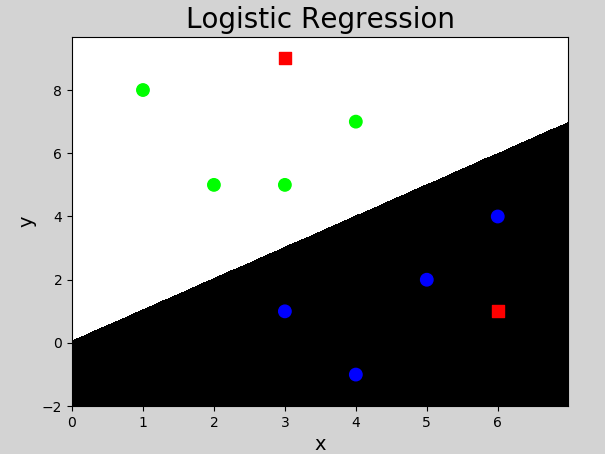## 多分类实现

$x_1$ $x_2$ $x_3$ A
$x_1$ $x_2$ $x_3$ B
$x_1$ $x_2$ $x_3$ C

……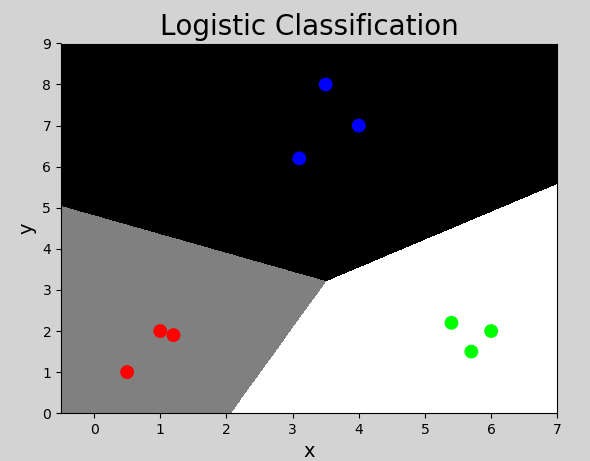## 总结

1）逻辑回归是分类问题，用于实现二分类问题

2）实现方式：利用线性模型计算，在逻辑函数作用下产生分类

3）多分类实现：可以将多分类问题转化为二分类问题实现

4）用途：广泛用于各种分类问题

# 决策树

## 什么是决策树

【示例1】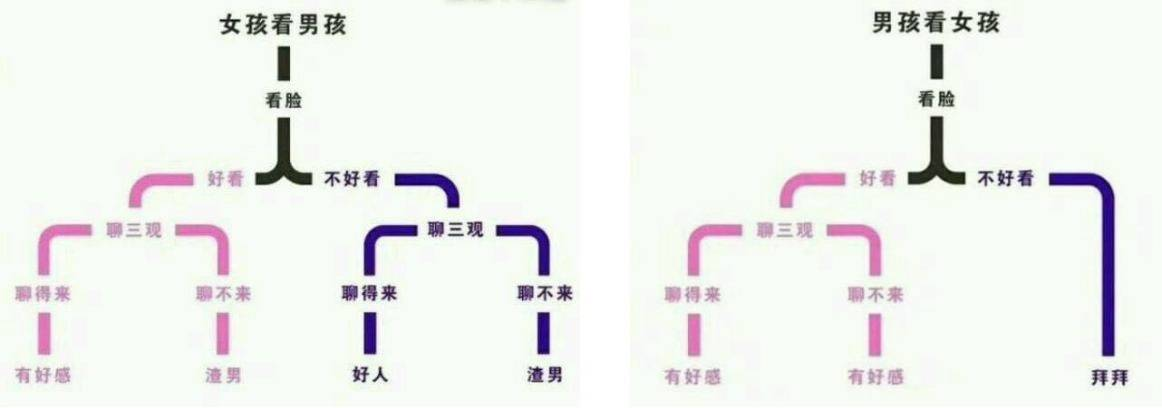【示例2】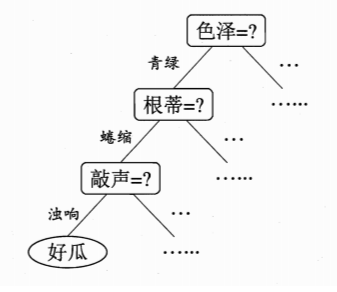## 决策树的结构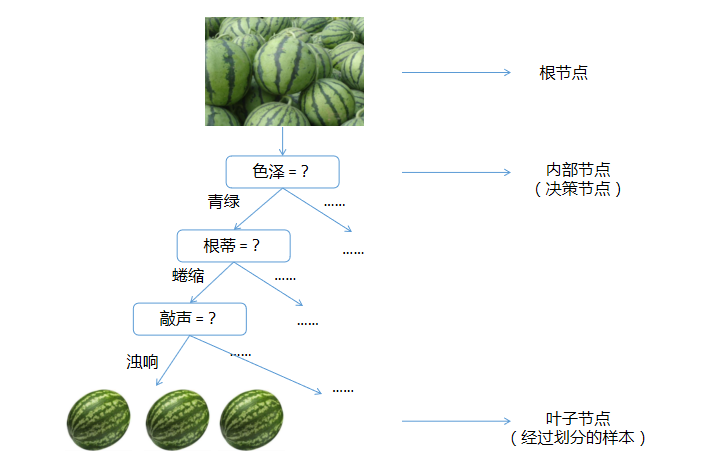## 如何构建决策树

### 构建决策树算法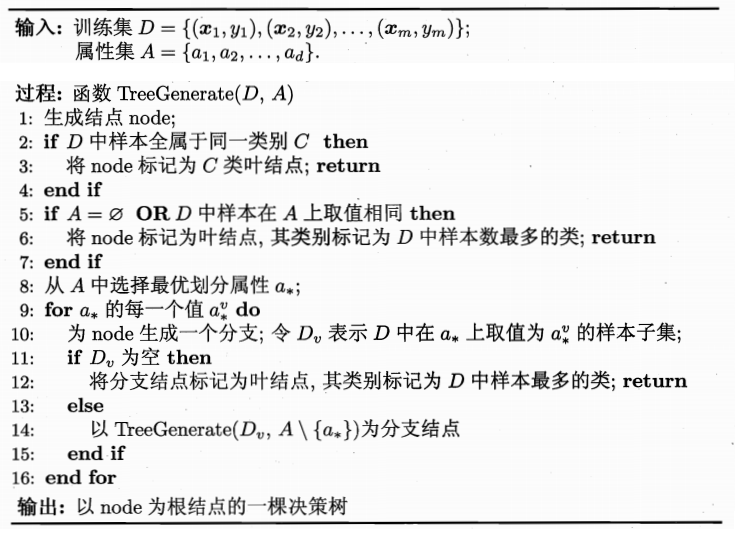• 如何选取特征. 决策树构建的每一步，应该挑选最优的特征，进行决策对数据集划分效果最好；
• 决定何时停止分裂子节点.

### 如何选择特征

#### ① 信息熵

$H = -\sum_{i=1}^{n}{P(x_i)log_2P(x_i)}$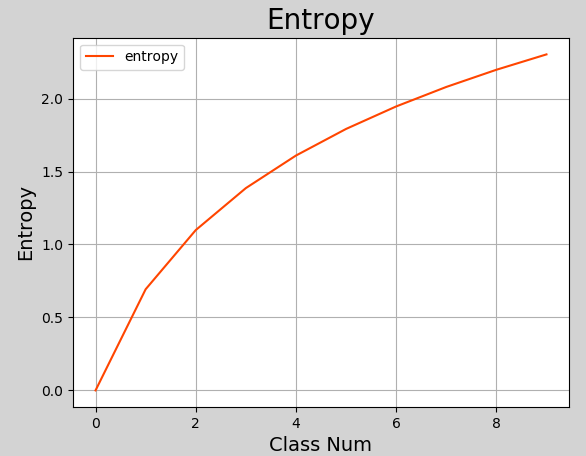#### ② 信息增益

$Gain(D, a) = Ent(D) - \sum_{v=1}^{V} \frac{|D^v|}{|D|} Ent(D^v)$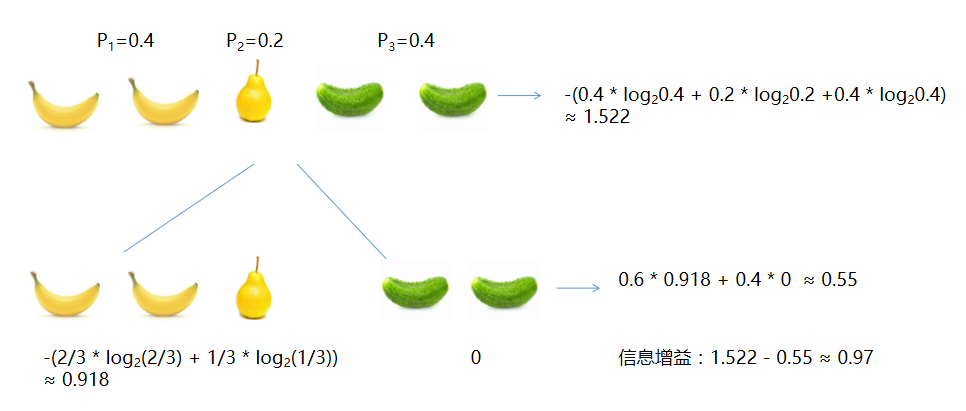• 香蕉占2/5，所以$P_1=0.4$；梨占1/5，所以$P_2 = 0.2$；黄瓜占1/5，所以$P_3 = 0.4$
• 根节点信息熵：$-(0.4 * log_2 0.4 + 0.2 * log_2 0.2 + 0.4 * log_2 0.4) \approx 1.522$
• 根据颜色划分后：黄色分支信息熵$-(\frac{2}{3} * log_2 \frac{2}{3} + \frac{1}{3} * log_2 \frac{1}{3}) \approx 0.918$；绿色分支信息熵$-(1.0 * log_2 1.0) = 0$；整个第二层信息熵为$0.6 * 0.918 + 0.4 * 0 \approx 0.55$
• 根据颜色划分后的信息增益：$1.522 - 0.55 \approx 0.97$

#### ③ 增益率

$Gain\_ratio(D, a) = \frac{Gain(D, a)}{IV(a)}$

$IV(a) = - \sum_{v=1}^{V} \frac{|D^v|}{|D|} log_2 \frac{|D^v|}{|D|}$

#### ④ 基尼系数

$Gini(p) = \sum_{k=1}^{k} p_k (1-p_k) = 1 - \sum_{k=1}^{k} p_k^2$

$Gini\_index(D, a) = \sum_{v=1}^{V} \frac{|D^v|}{|D|} Gini(D^v)$

### 如何停止分裂

• 当前节点所有样本属于同一个类别，无需划分
• 当前属性集为空，或者所有样本取值相同，无法划分
• 当前节点包含的样本集合为空，不能划分
• 当前节点样本数量少于指定数量

## 如何实现决策树

scikit-learn中决策树相关API：

【案例】波士顿房价预测

• 数据集介绍

该数据集为一个开放房价数据集，包含506笔样本，每个样本包含13个特征和1个标签，具体如下所示：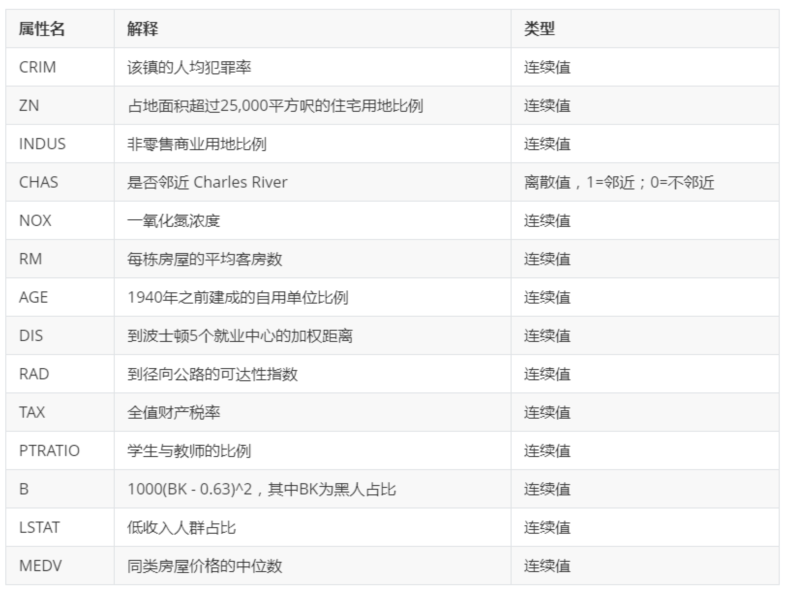• 代码实现
• 执行结果
• 特征重要性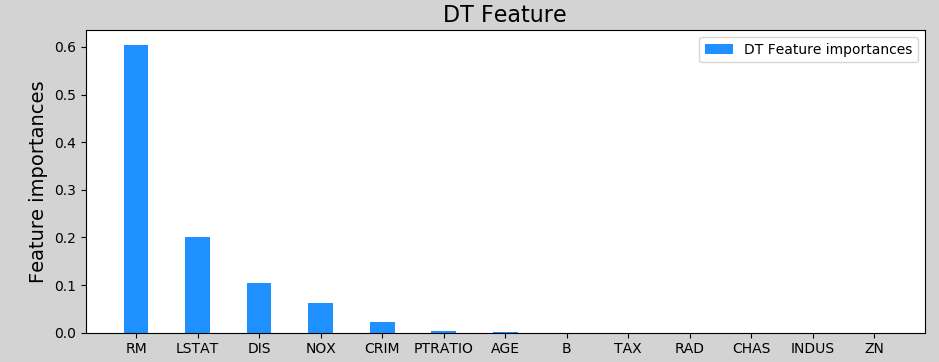## 决策树的剪枝

（1）预剪枝. 决策树生成过程中，对每个节点在划分前进行评估，若当前节点不能带来决策树泛化性能的提升，则停止划分并将当前节点标记为叶子节点.

（2）后剪枝. 先训练为一颗完整的决策树，然后自低向上对非叶子节点进行考察，若将该节点对应的子树替换为叶节点能带来决策树泛化能力提升，则将该子树替换为叶节点.

# 集成学习与随机森林

## 集成学习

• 个体间存在强依赖关系，必须串行生成的序列化方法，其代表为Boosting算法；
• 个体之间不存在强依赖关系，可同时生成的并行化方法，代表是Bagging和随机森林算法.

## Boosting

### 什么是Boosting

Boosting（直译为推进、提升）是一族可以将弱学习器提升为强学习器的算法，其工作原理是：

• 先训练出一个初始模型；
• 根据模型的表现进行调整，使得模型预测错误的数据获得更多的关注，再重新训练下一个模型；
• 不断重复第二步，直到模型数量达到预先设定的数目T，最终将这T个模型加权结合.

## 随机森林

### 如何实现随机森林

sklearn中，随机森林相关API：

# 总结

1）什么是决策树：利用样本特征进行决策归类，将具有相同属性的样本划入一个子节点

2）决策树的用途：用作分类器、回归器

3）如何构建决策树：根据信息增益、增益率、基尼系数构建

4）什么情况下使用决策树：实用性较广，课用于一般回归、分类问题

5）决策树优化：集成学习、随机森林

# 练习

（1）有一批水果（如下图所示），按照形状进行分类，将长条形、椭圆形各划分到不同子节点中，计算划分后的信息增益.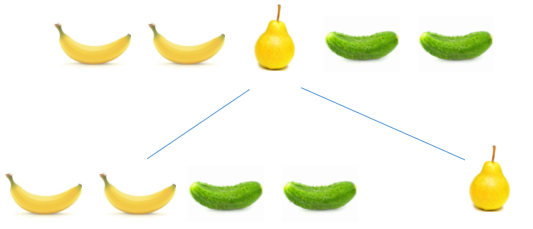（2）一批样本包含A和B两个类别，计算：当A类别比率依次占0%, 10%, 20%, …, 100%时，这批样本信息熵值，并以占比作为x轴数值、信息熵作为y轴数值绘制图像.

# 延伸阅读

• VMware公司使用定制的决策树进行定价优化

# 一、支持向量机

## 基本概念

### 什么是支持向量机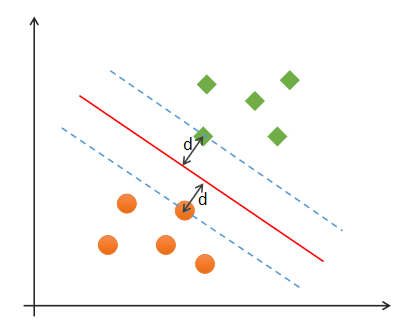### 最优分类边界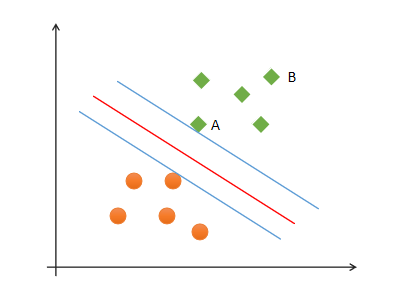$w^T x + b = 0$

$\gamma = \frac{1}{||w||}$

### SVM最优边界要求

SVM寻找最优边界时，需满足以下几个要求：

（1）正确性：对大部分样本都可以正确划分类别；

（2）安全性：支持向量，即离分类边界最近的样本之间的距离最远；

（3）公平性：支持向量与分类边界的距离相等；

（4）简单性：采用线性方程（直线、平面）表示分类边界，也称分割超平面。如果在原始维度中无法做线性划分，那么就通过升维变换，在更高维度空间寻求线性分割超平面. 从低纬度空间到高纬度空间的变换通过核函数进行。

### 线性可分与线性不可分

#### ② 线性不可分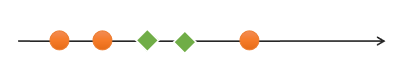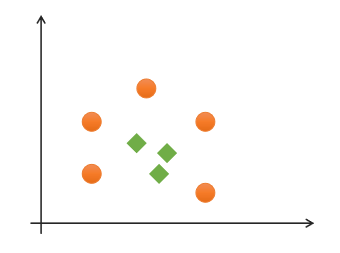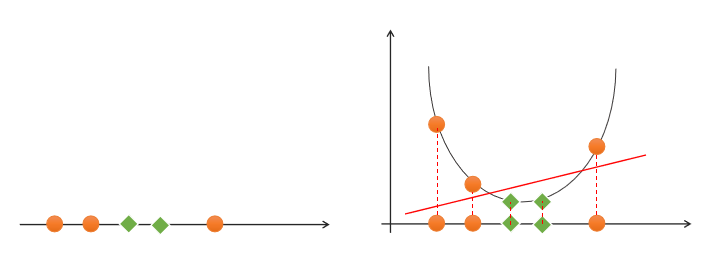![](https://image.discover304.top/ai/svm_6.png)

## 核函数

### 线性核函数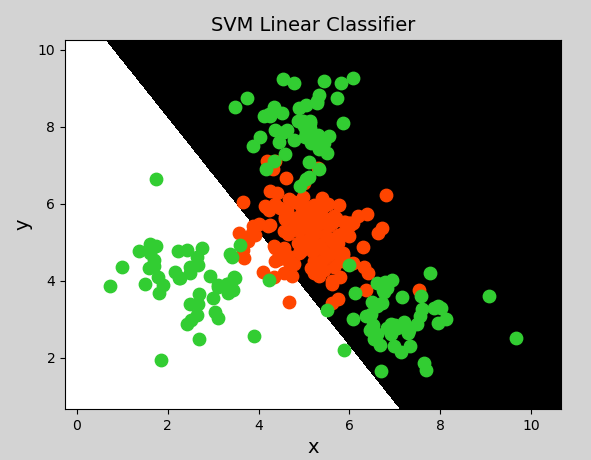### 多项式核函数

$K(x，y)=(αx^T·y+c)d$

$y = x_1 + x_2\\ y = x_1^2 + 2x_1x_2+x_2^2\\ y=x_1^3 + 3x_1^2x_2 + 3x_1x_2^2 + x_2^3$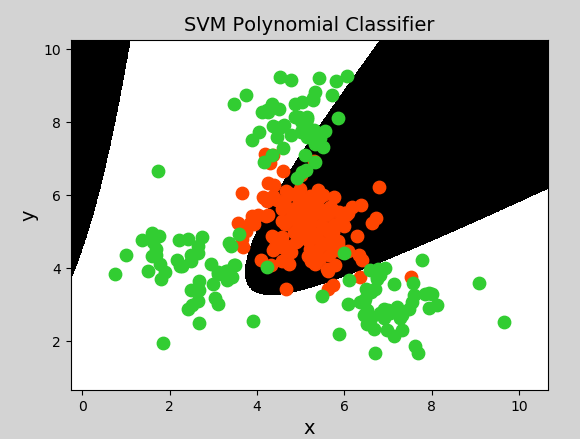### 径向基核函数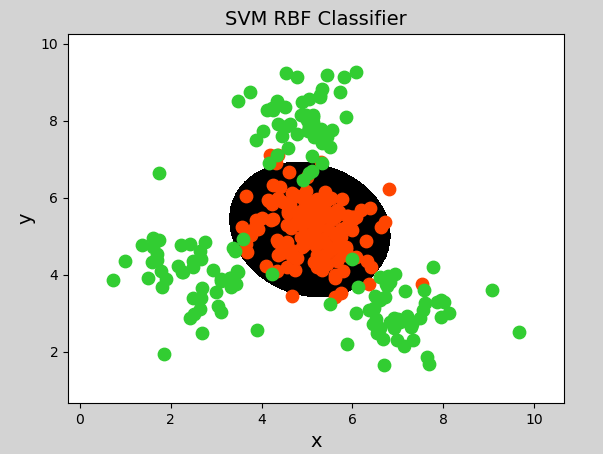## 总结

（1）支持向量机是二分类模型

（2）支持向量机通过寻找最优线性模型作为分类边界

（3）边界要求：正确性、公平性、安全性、简单性

（4）可以通过核函数将线性不可分转换为线性可分问题，核函数包括：线性核函数、多项式核函数、径向基核函数

（5）支持向量机适合少量样本的分类

# 朴素贝叶斯

## 概率

### 定义

（1）抛一枚硬币，可能正面朝上，可能反面朝上，这是随机事件. 正/反面朝上的可能性称为概率；

（2）掷骰子，掷出的点数为随机事件. 每个点数出现的可能性称为概率；

（3）一批商品包含良品、次品，随机抽取一件，抽得良品/次品为随机事件. 经过大量反复试验，抽得次品率越来越接近于某个常数，则该常数为概率.

### 联合概率与条件概率

p(下雨|阴天)

#### ③ 事件的独立性

$P(AB)=P(A)P(B)$

$P(A|B) = P(A)$

## 贝叶斯定理

### 定义

$P(A|B) = \frac{P(A)P(B|A)}{P(B)}$

$P(A,B) =P(B)P(A|B)\\ P(B,A) =P(A)P(B|A)$

$P(B)P(A|B)=P(A)P(B|A)$

### 贝叶斯定理示例

【示例一】计算诈骗短信的概率

$P(A|B) = P(A) P(B|A) / P(B) = 0.05 * 0.5 / 0.04 = 0.625$

【示例二】计算喝酒驾车的概率

$P(A|B) = P(A) P(B|A) / P(B) = 0.2 * 0.05 / 0.1 = 0.1$

p(女) = 0.4

p(裤子|女) = 0.5

p(裤子) = 0.8

P(女|裤子) = 0.4 * 0.5 / 0.8 = 0.25

$P(A|B) = \frac{P(A)P(B|A)}{P(B)}$

## 朴素贝叶斯分类器

### 分类原理

Text Category
A great game（一个伟大的比赛） Sports（体育运动）
The election was over（选举结束） Not sports（不是体育运动）
Very clean match（没内幕的比赛） Sports（体育运动）
A clean but forgettable game（一场难以忘记的比赛） Sports（体育运动）
It was a close election（这是一场势均力敌的选举） Not sports（不是体育运动）

$P(Sports | a \ very \ close \ game) = \frac{P(a \ very \ close \ game | sports) * P(sports)}{P(a \ very \ close \ game)}$

$P(Not \ Sports | a \ very \ close \ game) = \frac{P(a \ very \ close \ game | Not \ sports) * P(Not \ sports)}{P(a \ very \ close \ game)}$

$P(a \ very \ close \ game) = P(a) * P(very) * P(close) * P(game)$

$P（a \ very \ close \ game|Sports)= \\ P(a|Sports)*P(very|Sports)*P(close|Sports)*P(game|Sports)$

• 第一步：计算总词频：Sports类别词语总数14，Not Sports类别词语总数9

• 第二步：计算每个类别的先验概率

其中，分子部分加1，是为了避免分子为0的情况；分母部分都加了词语总数14，是为了避免分子增大的情况下计算结果超过1的可能.

• 第三步：将先验概率带入贝叶斯定理，计算概率：

是体育运动的概率：

$P（a \ very \ close \ game|Sports)= \\ P(a|Sports)*P(very|Sports)*P(close|Sports)*P(game|Sports)= \\ 0.12 * 0.08 * 0.04 * 0.12 = 0.00004608$

​ 不是体育运动的概率：

$P（a \ very \ close \ game|Not \ Sports)= \\ P(a|Not \ Sports)*P(very|Not \ Sports)*P(close|Not \ Sports)*P(game|Not \ Sports)= \\ 0.087 * 0.043 * 0.087 * 0.043 = 0.000013996$

### 实现朴素贝叶斯分类器

• GaussianNB（高斯朴素贝叶斯分类器）：适合用于样本的值是连续的，数据呈正态分布的情况（比如人的身高、城市家庭收入、一次考试的成绩等等）
• MultinominalNB（多项式朴素贝叶斯分类器）：适合用于大部分属性为离散值的数据集
• BernoulliNB（伯努利朴素贝叶斯分类器）：适合用于特征值为二元离散值或是稀疏的多元离散值的数据集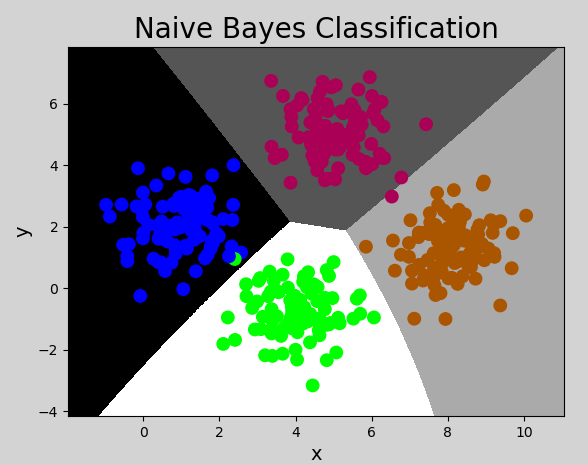## 总结

1）什么是朴素贝叶斯：朴素贝叶斯法是基于贝叶斯定理与特征条件独立假设的分类方法。“朴素”的含义为：假设问题的特征变量都是相互独立地作用于决策变量的，即问题的特征之间都是互不相关的。

2）朴素贝叶斯分类的特点

① 优点

• 逻辑性简单
• 算法较为稳定。当数据呈现不同的特点时，朴素贝叶斯的分类性能不会有太大的差异。
• 当样本特征之间的关系相对比较独立时，朴素贝叶斯分类算法会有较好的效果。

② 缺点

• 特征的独立性在很多情况下是很难满足的，因为样本特征之间往往都存在着相互关联，如果在分类过程中出现这种问题，会导致分类的效果大大降低。

3）什么情况下使用朴素贝叶斯：根据先验概率计算后验概率的情况，且样本特征之间独立性较强。